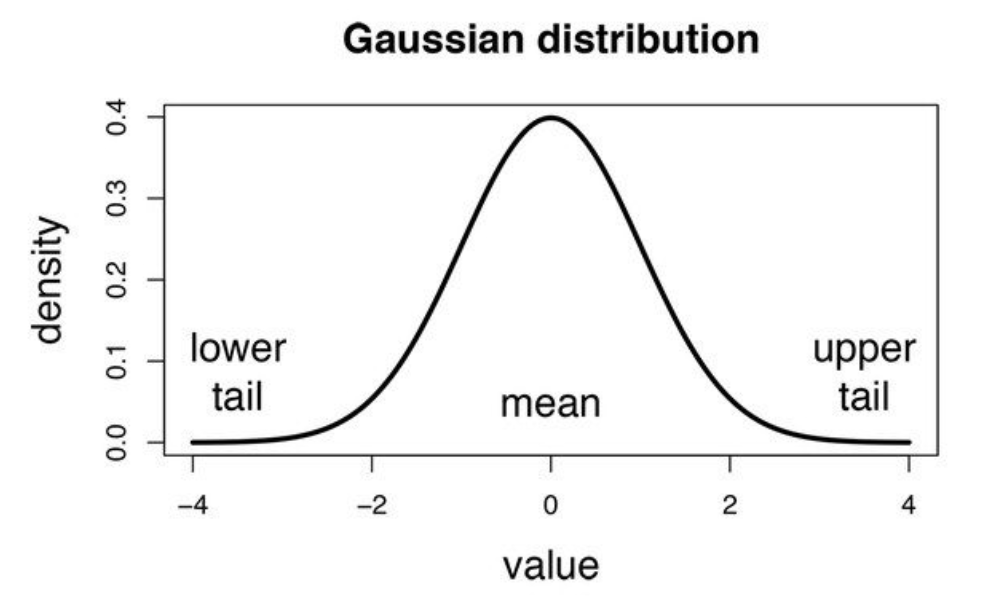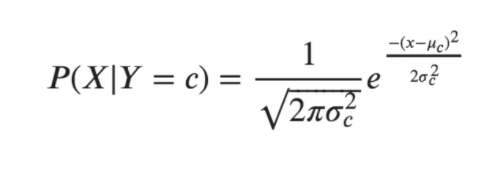# Gaussian Naive Bayes

## Introduction

Uncertainty is difficult for humans to bear. However, algorithms in machine learning can help you get over this limitation. That is where Naive Bayes kicks in. For predictive modeling, this is a basic but surprisingly strong algorithm. The probabilistic algorithm Naive Bayes is utilised in a number of classification tasks.

Naive Bayes models are further classified into three categories:

• Gaussian Naive Bayes
• Bernoulli
• Multinomial Naive Bayes

Before we get started, let's have a look at what Naive Bayes is all about. Also, To explain Naive Bayes, we need to get a glimpse of the Bayes theorem.

## Bayes theorem

The foundation of the Bayes theorem is conditional probability. In truth, the Bayes theorem is simply a different or reverse method of calculating conditional probability.

Let us define the different types of probabilities:

• Marginal Probability: The chance of an event occurring regardless of the outcomes of other random factors. e.g. P(A)
• Joint Probability: Probability of two or more simultaneous events.e.g., P(A and B) or P(A, B)
• Conditional Probability: Probability of one (or more) event given the occurrence of another event, e.g., P(A given B) or P(A | B).

Conditional probability is expressed as follows:

Also, the reverse for the same can be written similarly.

Therefore the final form we have is :

Where:

P(A|B) is known as posterior

P(B|A) is called the likelihood

P(A) is called the prior probability

P(B) is  marginal probability,

A more generalized for A1,sa2…A set of mutually exclusive and exhaustive events can be written as:

## Gaussian Naive Bayes

We've seen that the events(A1, A2,...) are in categories so far, but what about when an event is a continuous variable? If we suppose that event follows a specific distribution, the probability of likelihoods are calculated using the probability density function of that distribution.If we assume that events follow a Gaussian or normal distribution, we must use its probability density and call it Gaussian Naive Bayes.

For the Naive gaussian Bayes, we use the following form:The variance and mean of the continuous variable X determined for a given class c of Y are σ and μ in the following formulas.

Let's use Python,, and Scikit Learn to implement Gaussian Naive Bayes in practice.

## Implementation

``````%matplotlib inline

From sklearn.datasets import make_blobs, make_moons, make_regression, load_iris
from scipy.stats import multivariate_normal
import matplotlib.pyplot as plt
import numpy as np

# For the moment we only take a couple of features from the IRIS dataset for convenience of visualization

X = iris.data[:, 1:3]
Y = iris.target

print X.shape
print Y.shape
plt.scatter(X[:,0],X[:,1])

#algorithm implementation.

class BayesClassifier:

mu = None
cov = None
n_classes = None

def __init__(self):
a = None

def pred(self,x):
prob_vect = np.zeros(self.n_classes)

for i in range(self.n_classes):

mnormal = multivariate_normal(mean=bc.mu[i], cov=bc.cov[i])

# We use uniform priors
prior = 1./self.n_classes

prob_vect[i] = prior*mnormal.pdf(x)
sumatory = 0.
for j in range(self.n_classes):
mnormal = multivariate_normal(mean=bc.mu[j], cov=bc.cov[j])
sumatory += prior*mnormal.pdf(x)
prob_vect[i] = prob_vect[i]/sumatory
return prob_vect

def fit(self, X,y):
self.mu = []
self.cov = []

self.n_classes = np.max(y)+1

for i in range(self.n_classes):
Xc = X[y==i]

mu_c = np.mean(Xc, axis=0)
self.mu.append(mu_c)

cov_c = np.zeros((X.shape, X.shape))
for j in range( Xc.shape):
a = Xc[j].reshape((X.shape,1))
b = Xc[j].reshape((1,X.shape))
cov_ci = np.multiply(a, b)
cov_c = cov_c+cov_ci
cov_c = cov_c/float(X.shape)
self.cov.append(cov_c)
self.mu = np.asarray(self.mu)
self.cov = np.asarray(self.cov)

# Fit the classifier
bc = BayesClassifier()

bc.fit(X,Y)``````

## FAQs

1. Is Gaussian naive Bayes suitable for datasets with a large number of attributes??
If the number of characteristics is enormous, the computation cost will be considerable, and the Curse of Dimensionality will apply.

2. What is the zero frequency phenomenon?
The model is given a 0 (zero) probability if a category is not recorded in the training set but occurs in the test data set, resulting in an erroneous estimate.

3. What exactly does the word distribution signify?
Distribution shows how values disperse in series and how frequently they appear in this series.

4. When is gaussian naive bayes used?
It is more likely to be used when each class follows a Gaussian distribution.

5. What is a confusion matrix?
A confusion matrix is a method for evaluating machine learning classification performance. It allows you to assess the performance of the classification model using a set of test data for which the true and false values are known.

## Key Takeaways

There are several different  naive Bayes algorithms whose usability can be referred to here.